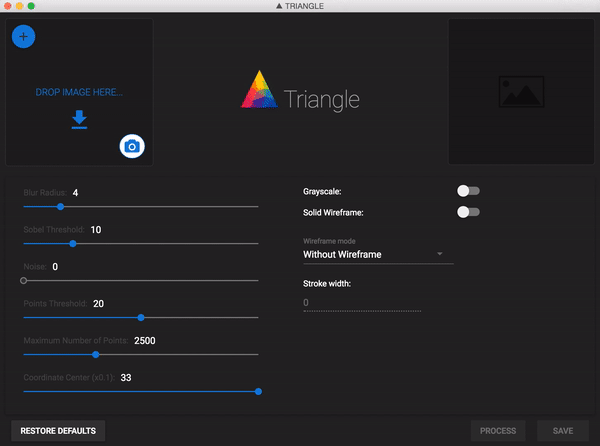﻿ Endre Simo - Delaunay image triangulation

# Delaunay image triangulation

Categories: Blog,Experiments,General,Generative,Go,Programming,Tutorials

In this article we present a technique to triangulate source images, converting them to abstract, someway artistic images composed of tiles of triangles. This will be an attempt to explore the fields between computer technology and art.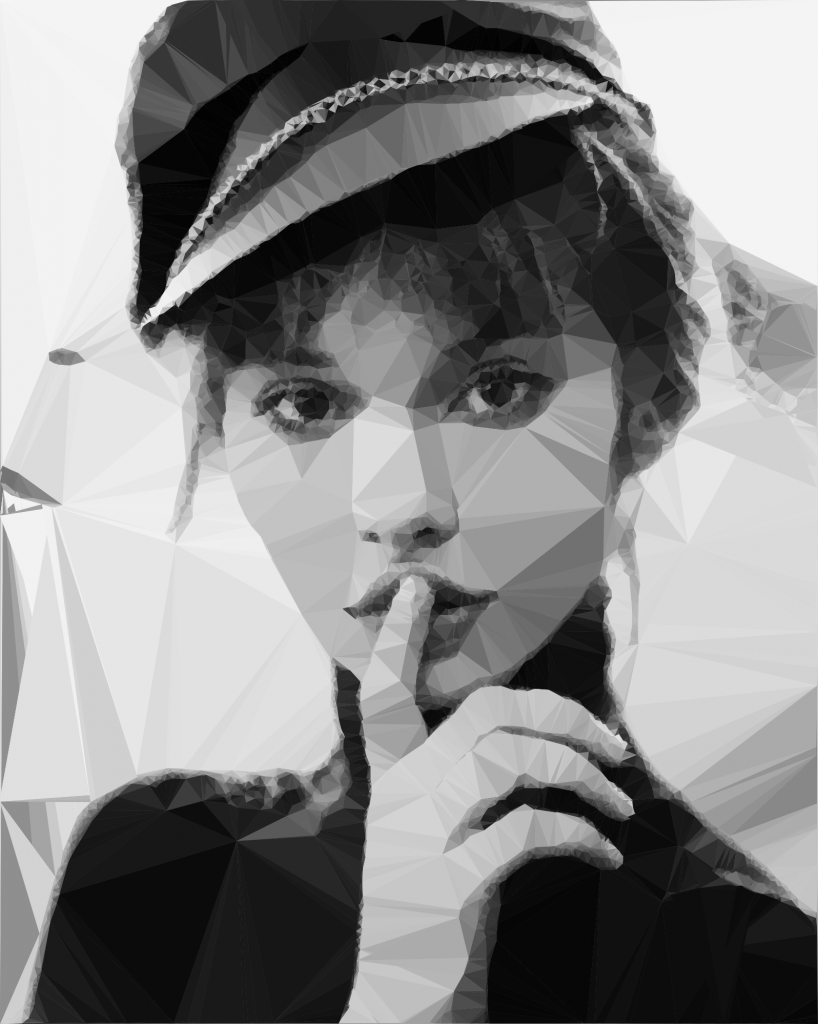But first, what is triangulation? Simply spoken a triangulation partitions a polygon into triangles which allows, for instance, to compute the area of a polygon and execute some operations on the computed polygon surface. In other terms the triangulation might be conceived as a geometric object defined by a point set, but what differentiates the polygons from a point set is the latter does not have an interior, except if we treat the point set as a convex hull/polygon. But to think of a point set as a convex polygon, the points from the interior of the convex hull should not be ignored completely. This is what differentiates the Delaunay triangulation from the other triangulation techniques.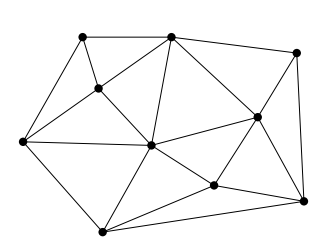Fig.1: Point set triangulation.

## Delaunay triangulation

Wikipedia has a very succinct definition of the Delaunay triangulation: ... a Delaunay triangulation (also known as a Delone triangulation) for a given set P of discrete points in a plane is a triangulation DT(P) such that no point in P is inside the circumcircle of any triangle in DT(P)

The circumcircle of a triangle is the unique circle passing through all the vertices of the triangle. This will get valuable meaning in the following. So considering that we have a set of six points we can obtain a triangulated polygon by tracing a circle around each vertex of the constructed triangle in such a manner that the circumcircle of all five triangles are empty. We also say, that the triangles satisfy the empty circle property (Fig.2).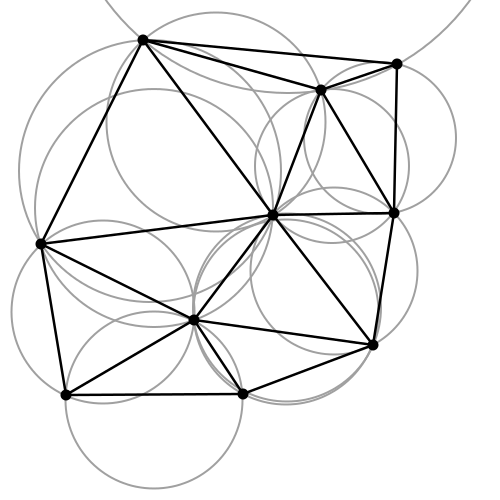Fig.2: All triangles satisfy the empty circle property.

After a short theoretical introduction now we want to apply this technique to a more practical but at the same time aesthetical field, concretely to transform a source image to it’s triangulated counterpart. Having the basic idea and it’s theoretical background we can start to construct the basic building blocks of the algorithm.

Above all a short notice: we use Go as programming language for the implementation. First, because it has a very clean and easy to use API, second because it can be well suited for a CLI based application, which single scope is to convert an input file to a destination object.

## The algorithm

Now into the algorithm. As the basic components are triangles we define a Triangle structs, having as constituents the nodes, it’s edges and the circumcircle which describes the triangle circumference.

``````
type Triangle struct {
Nodes  []Node
edges  []edge
circle circle
}
``````

Next we create the circumscribed circle of this triangle. The circumcircle of a triangle is the circle which has the three vertices of the triangle lying on its circumference. Once we have the circle center point we can calculate the distance between the node points and the triangle circumcircle. Then we can calculate the circle radius.

We can transpose this into the following Go code:

The question is how do we get the triangle points from the input image? To obtain a large triangle distribution on the image parts with more details we need somehow to analyze the image and mark the sensitive information. How do we do that? Sobel filter operator on the rescue. The Sobel operator is used in image processing to detect image edges. It’s working with an energy distribution matrix to differentiate the sensitive image informations from the less sensitive ones.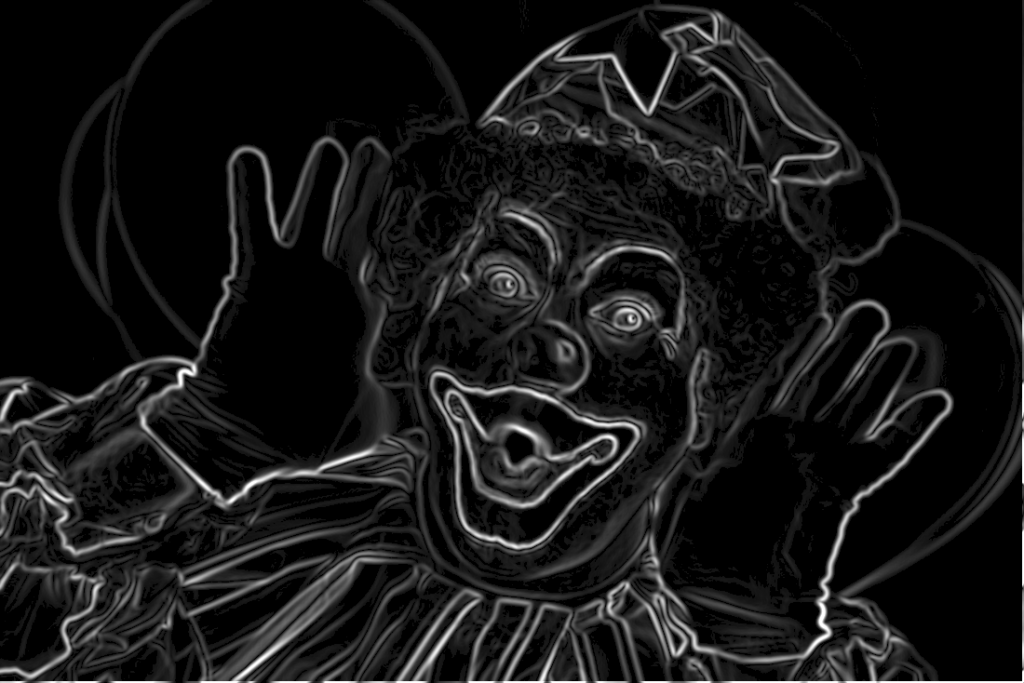Fig.3: Sobel operator applied to the source image.

Once we obtained the sobel image we can sparse the triangle points randomly by applying some threshold value. At the end we obtain an array of randomly distributed points, but these points are more dense on the sensitive image parts, and scarce on less sensitive ones.

Having the edge points we check whether the points are inside the triangle circumcircle or not. We save the triangle edges in case they are included and carry over otherwise. Then in a loop we search for (almost) identical edges and remove them.

Now that we have the nodes, in order to construct the triangulated image, the last thing we need to do is actually to draw the lines between nodes points by applying the underlying image pixel colors. The way we can achieve this is to loop over all the nodes and connect each edge point.

By tweaking the parameters we can obtain different kind of results. We can draw only the line strokes, we can apply different threshold values to filter out some edges, we can scale up or down the triangle sizes by defining the maximum point limit, we can export only to grayscale mode etc. The possibilities are endless.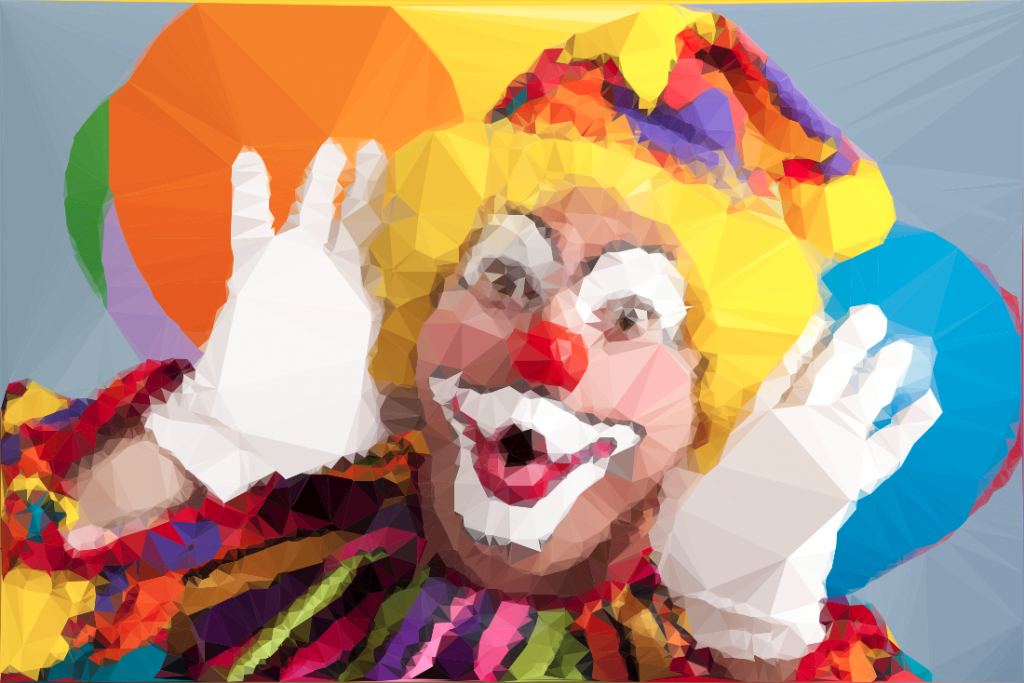Fig.4: Triangulated image example.

## Using Go interfaces to export the end result into different formats

By default the output is saved to an image file, but using interfaces, the Go way to achieve polymorphism, we can export even to a vector format. This is a nice touch considering that using a small image as input element we can export the result even to an svg file, which lately can be scaled up infinitely without image loss and consuming very low processing footprint.

The only thing we need to do is to declare an interface having a single method signature. This needs to be satisfied by each struct type implementing this method.

In our case we need two struct types, both of them implementing the same method differently. For example we could have an image struct type and an svg struct type declared in the following way:

Each of them will implement the same Draw method differently.

## Expose the algorithm as an API endpoint

Having a good system architecture, coupled with Go blazing fast execution time and goroutines (another feature of the Go language to parallelize the execution) the algorithm can be exposed to an API endpoint in order to process a large amount of images and then make it accessible through a web application.

The source code can be found on my Github page:

https://github.com/esimov/triangle

I have also created an Electron/React desktop application for everyone who do not want to be bothered with the terminal commands and needs something more user friendly.

The source file can also be found on my Github account: https://github.com/esimov/triangle-app